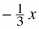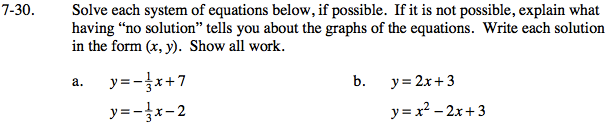### Home > GC > Chapter 7 > Lesson 7.1.3 > Problem7-30

7-30.
1. Solve each system of equations below, if possible. If it is not possible, explain what having “no solution” tells you about the graphs of the equations. Write each solution in the form (x, y). Show all work. Homework Help ✎

1. y =+ 7
y =− 2

2. y = 2x + 3
y = x2 − 2x + 3Use the Equal Values Method to solve.

$-\frac{1}{3}x+7=-\frac{1}{3}x-2$

7 ≠ -2

There is no solution because 7 ≠ -2. The lines are parallel, so there is no point of intersection.

Use the Equal Values Method to solve.

0 = x² - 4x

0 = x(x - 4)

Therefore, x = 0 or 4, and y = 2(0) + 3 = 3 or
y = 2(4) + 3 = 11.
So there are two points of intersection (0,3) and (4,11).